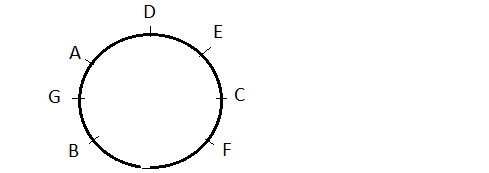## Reasoning Sitting ArrangementPage 2

###### Section-2
Read the following information carefully and answer the questions given below it.
A, B, C, D, E, F and G are playing cards sitting in a circle.
1. F is 2nd to the right of G.
2. B is neighbour of F but not of E.
3. E is neighbour of C, Who is 4th to the right of G.
4. D is between E and A.

1.

 Who is fourth to the left of G? (a)D (b)E (c)C (d)Cannot be determined
Answer is: CPerson C is fourth to the left of G.

2.

 Who is the left of G? (a)A (b)C (c)B (d)None of these
Answer is: APerson A is to thr left of G.

3.

 Who are the neighbours of F? (a)E and C (b)F and B (c)A and B (d)C and B
Answer is: DC and B are the neighbours of F.